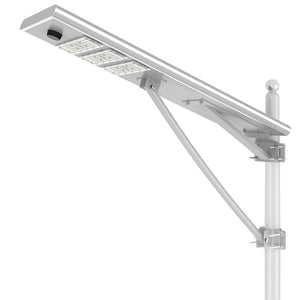# Why do you need solar lights?

June 17, 2021

A lumen is a unit of measurement indicating the visible light output of a light source. In practical terms, lumens tell you how bright a light bulb is. Light bulbs and some lighting fixtures carry a specific lumen value. The higher the lumens, the brighter the bulb. Lighting designers often use lumens per square foot to ensure a home has sufficient lighting. The basic calculation is very simple:

• Lumens per square foot = total lumens divided by the total square footage of the area.

You can use this calculation to determine how much light per square foot you currently have in a room or to find out how much light you need (or should have) in a given area.

## Measuring Square Footage

Square footage is the same thing as wall-to-wall floor space. To measure the square footage of a room, measure the width and the length of the room, then multiply the numbers. For example, a 10-foot-wide, 12-foot-long room is 120 square feet (10 x 12 = 120).

## Calculating Lumens

If you're curious to know how much light a room is getting, simply add up the lumens of all the light bulbs in the room, and then divide by the room's square footage to find the lumens per square foot. For example, for a 120-square-foot room with a single overhead fixture with two light bulbs, each producing 800 lumens:

• 800 + 800 = 1,600 lumens
• 1,600/120 = 13.3 lumens per square foot.

Finding the lumens is easy. Most energy-efficient (LED and fluorescent) light bulbs have their lumens value printed right on the bulb base. Incandescent bulbs list the lumens on the bulb packaging; if you can't find that, look for the wattage rating on the bulb's base and convert that to lumens using a wattage–lumens chart.